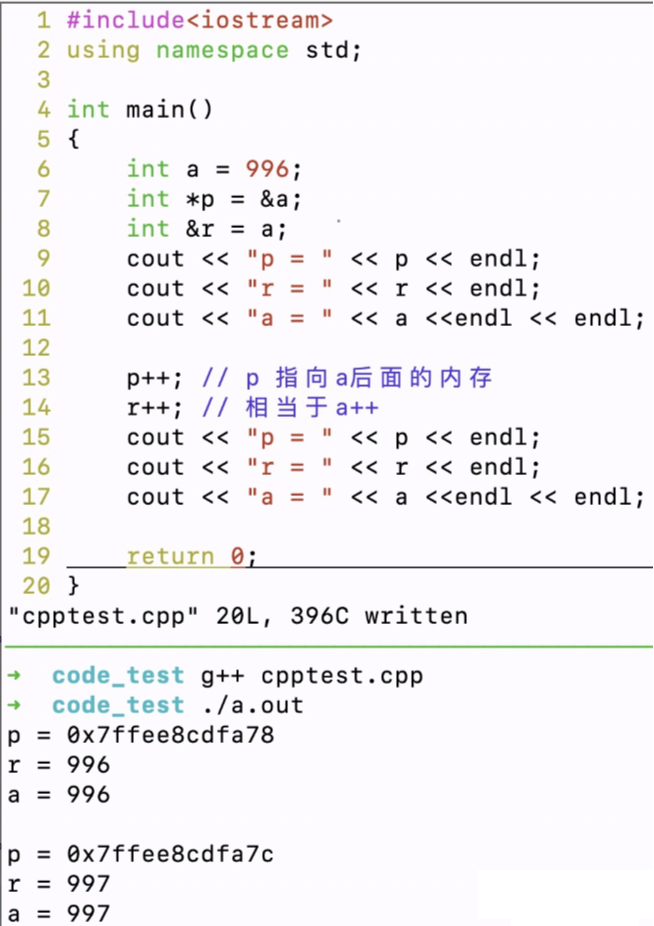• 2
回复
• 收藏
• 点赞
• 分享
• 发新帖
• c++ 引用和指针区别

# c++ 引用和指针区别

## 1、两者的定义和性质不同

``````int a = 996;
int *p = &a; // p是指针, &在此是求地址运算
int &r = a; // r是引用, &在此起标识作用``````

## 2、指针可以有多级，引用只能是一级

``````int **p; // 合法
int &&a; // 不合法``````

## 3、指针可以在定义的时候不初始化，引用必须在定义的时候初始化

``````int *p; // 合法
int &r; // 不合法
int a = 996;
int &r = a; // 合法``````

## 4、指针可以指向NULL，引用不可以为NULL

``````int *p = NULL; // 合法
int &r = NULL; // 不合法``````

## 5、指针初始化之后可以再改变，引用不可以

``````int a = 996;
int *p = &a; // 初始化, p 是 a 的地址
int &r = a; // 初始化, r 是 a 的引用

int b = 885;
p = &b;	// 合法, p 更改为 b 的地址
r = b; 	// 不合法, r 不可以再变``````

## 6、sizeof 的运算结果不同

``````int a = 996;
int *p = &a;
int &r = a;

cout << sizeof(p); // 返回 int* 类型的大小
cout << sizeof(r); // 返回 int 类型的大小``````

sizeof 是C/C++ 中的一个操作符（operator），其作用就是返回一个对象或者类型所占的内存字节数。

## 7、自增运算意义不同## 8、指针和引用作为函数参数时，指针需要检查是否为空，引用不需要

``````void fun_p(int *p)
{
// 需要检查P是否为空
if (p == NULL)
{
// do something
}
}

void fun_r(int &r)
{
// 不需要检查r
// do something
}``````

## 使用引用的意义和作用：

1.  引用作为函数的参数，其作用与指针作为函数参数相似，函数对形参的操作，等于对实参本身进行操作；

2.  函数调用时，系统采用值传递的方式将实参的值传递给形参，系统会在内存中开辟空间来存储形参变量，并将实参的值复制给形参， 而采用引用作为函数形参，只要传给函数实参的别名，不需要耗费时间在内存中开辟空间存储形参，使用引用，可以提高函数的时间效率，并节省内存空间。

3. C++中推荐使用引用而非指针作为函数的参数，指针作为函数形参变量时，调用函数时仍需要在内存中分配空间。

4. C++的数组类型是带有长度信息的，引用传递时 如果是数组必须指定数组的长度。

``````#include <iostream>
using namespace std;

// 函数声明
void swap(int& x, int& y);

int main ()
{
// 局部变量声明
int a = 100;
int b = 200;

cout << "交换前，a 的值：" << a << endl;
cout << "交换前，b 的值：" << b << endl;

/* 调用函数来交换值 */
swap(a, b);

cout << "交换后，a 的值：" << a << endl;
cout << "交换后，b 的值：" << b << endl;

return 0;
}

// 函数定义
void swap(int& x, int& y)
{
int temp;
temp = x; /* 保存地址 x 的值 */
x = y;    /* 把 y 赋值给 x */
y = temp; /* 把 x 赋值给 y  */

return;
}

## 指针的意义和作用：

lilipo
LV.2
2
03-13 11:41

0

05-07 14:05

0